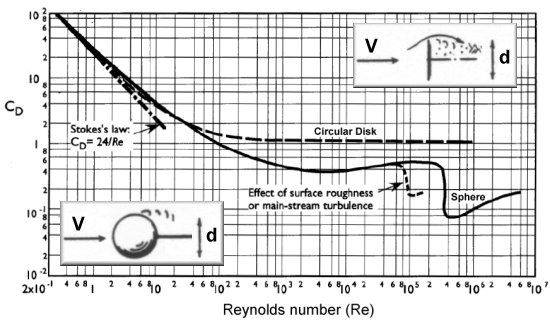# Equations for a mass falling to Earth from a distance

shibe
I have a question :
If we consider the change in g due to distance from the Earth core; then

y=distance from earth’s core
t=time
G=gravitation constant
M=Earth’s mass
k=GM

$$y^2(t)=\frac{k}{y(t)^2}$$

If we consider air resistive force as proportional to speed squared, then:

m=falling object mass
$$y^2(t)=\frac{k}{y(t)^2}-by’(t)^2$$

And if we go even further beyond:
Then we know that the air density changes depending on the distance of the falling object from the Earth which would affect the drag coefficient, so the constant b is a function of y.

So we have the second order, non linear differential equation :

$$y^2(t)=\frac{k}{y(t)^2}-b(y)y’(t)^2$$

So my question is,

0.) what is the precise relationship between the constant b and distance from the Earth core ?

1.) how to experimentally determine drag coefficient ?

2) how to solve the given differential equation?

3.) *HOW TO IMPROVE THE MODEL EVEN FURTHER* ?

Last edited:

DrStupid
$$y^2(t)=\frac{k}{y(t)^2}-by(t)^2$$

You are using the symbol ##y## for both, distance and speed.

$$y^2(t)=\frac{k}{y(t)^2}-b(y)y(t)^2$$

##b## also depends on speed.

0.) what is the precise relationship between the constant b and distance from the Earth core ?

For the density you may use the International Standard Atmosphere. For the drag coefficient see 1.)

1.) how to experimentally determine drag coefficient ?

With a wind tunnel or with numeric simulations.

2) how to solve the given differential equation?

Numerically

3.) *HOW TO IMPROVE THE MODEL EVEN FURTHER* ?

•shibe and PeroK
shibe
[ thanks for the corrections and link to ISA ]

how does b depend on speed
For air resistance proportional to v^2 ,
The Eqn is $$F_R=\frac{dA}{2}v^2$$

F_R=Resistive force
d=Fluid density
A=surface area
So $$b=\frac{dA}{2}$$

Also what do you mean by add extra “dimensions”

DrStupid
how does b depend on speed
That's complicate and depends on shape and surface properties. Here is an example for spheres and discs:(The Reynolds number is proportional to speed.)

Also what do you mean by add extra “dimensions”

Your equation is one-dimensional but the space we live in has three dimensions.

•shibe